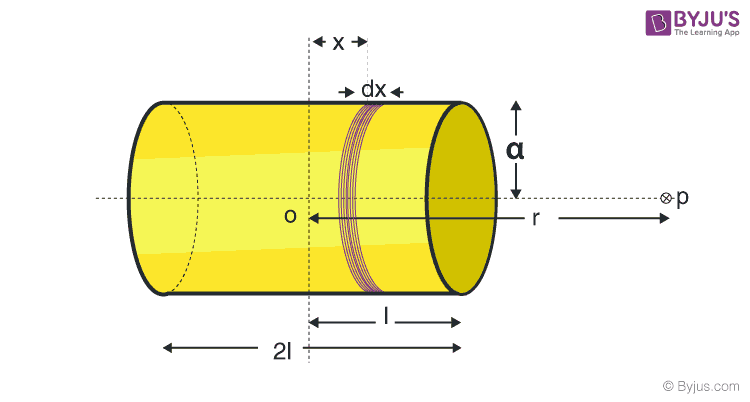# Bar Magnet As An Equivalent Solenoid

In order to understand how a bar magnet is equivalent to a solenoid, it becomes important to understand what is a bar magnet and what is a solenoid and what are their similarities and differences. In this article, let us learn about the derivation of a bar magnet as an equivalent solenoid.

## What is a Bar Magnet?

A bar magnet is a rectangular or a square piece of an object made from iron or steel having permanent magnetic properties with two poles: north and south.

## What is Solenoid?

A solenoid is a coil with a length greater than its diameter and is a type of electromagnet to produce controlled magnetic fields by passing an electric current through it.

You may also want to check out these topics given below!

## Derivation of Bar Magnet as an Equivalent Solenoid

By calculating the axial field of a finite solenoid carrying current, a bar magnet can be demonstrated as a solenoid. Consider a solenoid of radius a and length 2l with n number of turns per unit length that has current I passing through the solenoid. Considering a small element of thickness dx of the solenoid at distance x from O such that OP = r.Bar magnet as an Equivalent Solenoid

Magnetic field due to n turns at axis of solenoid

$$\begin{array}{l}dB=\frac{\mu _{0}ndxIa^{2}}{2\left [ (r-x)^{2}+a^{2} \right ]^{\frac{3}{2}}}\end{array}$$

Integrating x from -I to +I to get the magnitude of the total field

$$\begin{array}{l}B=\frac{\mu _{0}nIa^{2}}{2}\int_{-I}^{I}\frac{dx}{\left [ (r-x)^{2}+a^{2} \right ]^{\frac{3}{2}}}\end{array}$$

$$\begin{array}{l}\left [ (r-x)^{2} \right ]^{\frac{3}{2}}=r^{3}\end{array}$$

And

$$\begin{array}{l}B=\frac{\mu _{0}nIa^{2}}{2r^{3}}\int_{-I}^{I}dx\end{array}$$

$$\begin{array}{l}=\frac{\mu _{0}nI}{2}\frac{2Ia^{2}}{r^{3}}\end{array}$$

Therefore,

$$\begin{array}{l}B=\frac{\mu _{0}}{4\pi }\frac{2m}{r^{3}}\end{array}$$

From the above expression, it is understood that the magnetic moment of a bar magnet is equal to the magnetic moment of a solenoid.

### Difference between a Bar Magnet and a Solenoid

• The bar magnet is a permanent magnet whereas a solenoid is an electromagnet ie, it acts as a magnet only when an electric current is passed through.
• When a bar magnet is cut into two halves, both the pieces act as a magnet with the same magnetic properties whereas when a solenoid is cut into two halves, they will have weaker fields.
• The poles of the bar magnet are fixed whereas for a solenoid the poles can be altered.
• The strength of the magnetic field of a bar magnet is fixed ie, unaltered whereas the strength of the magnetic field of a solenoid depends on the electric current that is passed through it.

### Similarities between a Bar Magnet and a Solenoid

• Bar magnet and solenoid both have attractive and directive properties ie, to align itself along the external magnetic field.
• The magnetic field at the axial point is the same for both.
• The magnetic moment is the same for both.

### How Does A Solenoid Behave Like a Magnet?

Can you determine the north and south poles of a current-carrying solenoid with the help of a bar magnet?

Solution:

• A solenoid is a long coil of circular loops of insulated copper wire. Magnetic field lines are produced around the solenoid when a current is allowed to flow through it. The magnetic field produced by it is similar to the magnetic field of a bar magnet.
• The field lines produced in a current-carrying solenoid is shown in the above figure. When the north pole of a bar magnet is brought near the end connected to the negative terminal of the battery, the solenoid repels the bar magnet.
• Since like poles repel each other, the end connected to the negative terminal of the battery behaves as the North Pole of the solenoid and the other end behaves as the South Pole. Hence, one end of the solenoid behaves as the North Pole and the other end behaves as the South Pole.

Click on the video below, to know more about magnetism in detail.## Frequently Asked Questions – FAQs

### Is a bar magnet an electromagnet?

A bar magnet is not an electromagnet. It is a permanent magnet which gets magnetised and creates its own persistent magnetic field.

### What are the types of magnets?

The following are the types of magnets:
• Permanent Magnet
• Temporary Magnet
• Electromagnets

### List the uses of electromagnets.

Uses of electromagnets are:
• They are used in speakers, electric bells and buzzers.
• Electromagnets finds its application in relay, motors, generators and transformers.
• They are also used in MRI machines.

True.

### Which material is used in making a bar magnet.

Usually, materials like iron or steel are used in making a bar magnet.

Hope you learned about bar magnet as an equivalent solenoid in detail. To learn Physics concepts with the help of interactive video lessons, download BYJU’S – The Learning App!!.

Test your knowledge on Bar magnet as solenoid